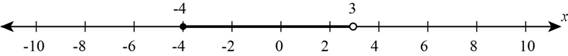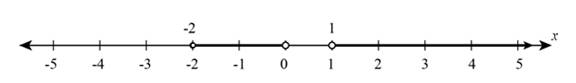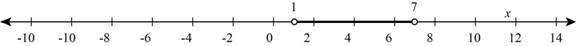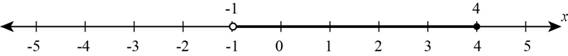# The solution of inequality − 4 &lt; 5 − 3 x ≤ 17 in interval notation and sketch the solution on real number line.### Precalculus: Mathematics for Calcu...

6th Edition
Stewart + 5 others
Publisher: Cengage Learning
ISBN: 9780840068071### Precalculus: Mathematics for Calcu...

6th Edition
Stewart + 5 others
Publisher: Cengage Learning
ISBN: 9780840068071

#### Solutions

Chapter 1, Problem 11T

(a)

To determine

## To evaluate: The solution of inequality −4<5−3x≤17 in interval notation and sketch the solution on real number line.

Expert Solution

The solution of inequality 4<53x17 is the interval [4,3) .

### Explanation of Solution

Given:

The inequality is 4<53x17 .

Calculation:

Section1:

Subtract same quantity from each side gives an equivalent inequalitythat is,

ABACBC

The solution of given inequality is all x-values that satisfy both the inequalities 4<53x and 53x17 so use above rule of inequality and subtract 5 from both the inequality,

45<3x1759<3x12

Multiply each side of inequality by negative quantity that is (1) which reverse the direction of inequality,

123x<9123x<934x<3

The set of solution consists all x-values from x=4 to x=3 include the endpoint 4 because inequality is satisfy at the endpoint 4 so the interval notation of inequality is [4,3) .

Thus,the solution of inequality 4<53x17 is the interval [4,3) .

Section2:

The solution of inequality from section 1 is [4,3) so graph of inequality is shown below,Figure (1)

Figure (1) shows the solution of inequality which includes all x-values from x=4 to x=3 and close interval at 4 and open interval at 3.

(b)

To determine

### To evaluate: The solution of inequality x(x−1)(x+2)>0 in interval notation and sketch the solution on real number line.

Expert Solution

The solution of inequality x(x1)(x+2)>0 is the interval (2,0)(1,) .

### Explanation of Solution

Given:

The inequality is x(x1)(x+2)>0 .

Calculation:

Section1:

The factors of the left-hand side are x, (x1) , (x+2) and these are zero when x=2,0,1 .

These values of x divide the real line into the intervals (,2) , (2,0) , (0,1) and (1,) .

Now, make a table indicating the sign of each factor on each interval,

 x<−2 −21 x − − + + (x−1) − − − + (x+2) − + + + x(x−1)(x+2) − + − +

From the above sign table, the inequality is satisfied on the intervals (2,0) , (1,) and the end point of intervals does not satisfy the inequality.

Thus, the solution of inequality x(x1)(x+2)>0 is the interval (2,0)(1,) .

Section 2:

The given inequality is x(x1)(x+2)>0 .

The solution of inequality from section 1 is (2,0)(1,) so graph of inequality is shown below,Figure (2)

Figure (2) shows the solution of inequality which includes all x-values from x=2 to x=0 and all values after x=1 because these x-coordinates does not satisfy the inequality they shows open interval on real line number.

(c)

To determine

### To evaluate: The solution of inequality |x−4|<3 in interval notation and sketch the solution on real number line.

Expert Solution

The solution of inequality |x4|<3 is the interval (1,7) .

### Explanation of Solution

Given:

The inequality is |x4|<3 .

Calculation:

Section1:

The inequality |x4|<3 is equivalent to,

3<x4<3

The solution of above inequality is all x-values that satisfy both the inequalities 3<x4 and x4<3 so add 4 to each side of inequality to get solution,

3+4<x<3+41<x<7

Thus, the solution of inequality |x4|<3 is the interval (1,7) .

Section2:

The solution of inequality from section 1 is (1,7) so graph of inequality is shown below,Figure (3)

Figure (3) shows the solution of inequality which includes all x-values from x=1 to x=7 and the endpoints does not satisfy the inequality so inequality shows open interval at both the sides on real number line.

(d)

To determine

### To evaluate: The solution of inequality 2x−3x+1≤1 in interval notation and sketch the solution on real number line.

Expert Solution

The solution of inequality 2x3x+11 is the interval (1,4] .

### Explanation of Solution

Given:

The inequality is 2x3x+11 .

Calculation:

Section1:

First move all terms to the left-hand side of the inequality then factor the inequality to get values of x,

2x3x+1102x3(x+1)x+10x4x+10

The factor of numerator is (x4) and denominator is (x+1) and these are zero when x=1,4 .

These values of x divide the real line into the intervals (,1) , (1,4) , and (4,) .

Now, make a table indicating the sign of each factor on each interval,

 x<−1 x=−1 −14 (x−4) − − − 0 + (x+1) − 0 + + + x−4x+1 + undefined − 0 +

From the sign table, the inequality is satisfied on the interval (1,4) and the end point 1 does not satisfy the inequality, endpoint 4 satisfy the inequality.

Thus, the solution of inequality 2x3x+11 is the interval (1,4] .

Section2:

The given inequality is 2x3x+11 .

The solution of inequality from section 1 is (1,4] so graph of inequality is shown below.Figure (4)

Figure (4) shows the solution of inequality which includes all x-values from x=1 to x=4 because these x-coordinates 1 does not satisfy the inequality they shows open interval and 4 satisfy the inequality they shows close interval on real number line.

### Have a homework question?

Subscribe to bartleby learn! Ask subject matter experts 30 homework questions each month. Plus, you’ll have access to millions of step-by-step textbook answers!